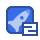# Probability for Machine Learning Discover How To Harness Uncertainty With Python10收藏

Probability is the bedrock of machine learning. You cannot develop a deep understanding and application of machine learning without it. Cut through the equations, Greek letters, and confusion, and discover the topics in probability that you need to know. Using clear explanations, standard Python libraries, and step-by-step tutorial lessons, you will discover the importance of probability to machine learning, Bayesian probability, entropy, density estimation, maximum likelihood, and much more.

...展开详情

• 5
资源
• 1
粉丝
•等级
•分享小兵Probability for Machine Learning Discover How To Harness Uncertainty With Python 9积分/C币 立即下载
1/3199积分/C币 立即下载